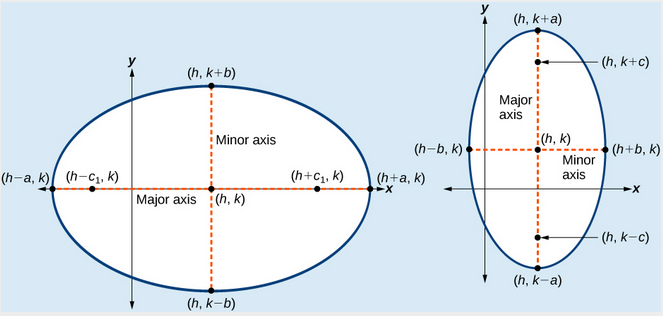## Writing Equations of Ellipses Not Centered at the Origin

Like the graphs of other equations, the graph of an ellipse can be translated. If an ellipse is translated $h$ units horizontally and $k$ units vertically, the center of the ellipse will be $\left(h,k\right)$. This translation results in the standard form of the equation we saw previously, with $x$ replaced by $\left(x-h\right)$ and y replaced by $\left(y-k\right)$.

### A General Note: Standard Forms of the Equation of an Ellipse with Center (h, k)

The standard form of the equation of an ellipse with center $\left(h,\text{ }k\right)$ and major axis parallel to the x-axis is

$\frac{{\left(x-h\right)}^{2}}{{a}^{2}}+\frac{{\left(y-k\right)}^{2}}{{b}^{2}}=1$

where

• $a>b$
• the length of the major axis is $2a$
• the coordinates of the vertices are $\left(h\pm a,k\right)$
• the length of the minor axis is $2b$
• the coordinates of the co-vertices are $\left(h,k\pm b\right)$
• the coordinates of the foci are $\left(h\pm c,k\right)$, where ${c}^{2}={a}^{2}-{b}^{2}$.

The standard form of the equation of an ellipse with center $\left(h,k\right)$ and major axis parallel to the y-axis is

$\frac{{\left(x-h\right)}^{2}}{{b}^{2}}+\frac{{\left(y-k\right)}^{2}}{{a}^{2}}=1$

where

• $a>b$
• the length of the major axis is $2a$
• the coordinates of the vertices are $\left(h,k\pm a\right)$
• the length of the minor axis is $2b$
• the coordinates of the co-vertices are $\left(h\pm b,k\right)$
• the coordinates of the foci are $\left(h,k\pm c\right)$, where ${c}^{2}={a}^{2}-{b}^{2}$.

Just as with ellipses centered at the origin, ellipses that are centered at a point $\left(h,k\right)$ have vertices, co-vertices, and foci that are related by the equation ${c}^{2}={a}^{2}-{b}^{2}$. We can use this relationship along with the midpoint and distance formulas to find the equation of the ellipse in standard form when the vertices and foci are given.Figure 7. (a) Horizontal ellipse with center $\left(h,k\right)$ (b) Vertical ellipse with center $\left(h,k\right)$

### How To: Given the vertices and foci of an ellipse not centered at the origin, write its equation in standard form.

1. Determine whether the major axis is parallel to the x– or y-axis.
1. If the y-coordinates of the given vertices and foci are the same, then the major axis is parallel to the x-axis. Use the standard form $\frac{{\left(x-h\right)}^{2}}{{a}^{2}}+\frac{{\left(y-k\right)}^{2}}{{b}^{2}}=1$.
2. If the x-coordinates of the given vertices and foci are the same, then the major axis is parallel to the y-axis. Use the standard form $\frac{{\left(x-h\right)}^{2}}{{b}^{2}}+\frac{{\left(y-k\right)}^{2}}{{a}^{2}}=1$.
2. Identify the center of the ellipse $\left(h,k\right)$ using the midpoint formula and the given coordinates for the vertices.
3. Find ${a}^{2}$ by solving for the length of the major axis, $2a$, which is the distance between the given vertices.
4. Find ${c}^{2}$ using $h$ and $k$, found in Step 2, along with the given coordinates for the foci.
5. Solve for ${b}^{2}$ using the equation ${c}^{2}={a}^{2}-{b}^{2}$.
6. Substitute the values for $h,k,{a}^{2}$, and ${b}^{2}$ into the standard form of the equation determined in Step 1.

### Example 2: Writing the Equation of an Ellipse Centered at a Point Other Than the Origin

What is the standard form equation of the ellipse that has vertices $\left(-2,-8\right)$ and $\left(-2,\text{2}\right)$ and foci $\left(-2,-7\right)$ and $\left(-2,\text{1}\right)?$

### Solution

The x-coordinates of the vertices and foci are the same, so the major axis is parallel to the y-axis. Thus, the equation of the ellipse will have the form

$\frac{{\left(x-h\right)}^{2}}{{b}^{2}}+\frac{{\left(y-k\right)}^{2}}{{a}^{2}}=1$

First, we identify the center, $\left(h,k\right)$. The center is halfway between the vertices, $\left(-2,-8\right)$ and $\left(-2,\text{2}\right)$. Applying the midpoint formula, we have:

$\begin{array}{l}\left(h,k\right)=\left(\frac{-2+\left(-2\right)}{2},\frac{-8+2}{2}\right)\hfill \\ \text{ }=\left(-2,-3\right)\hfill \end{array}$

Next, we find ${a}^{2}$. The length of the major axis, $2a$, is bounded by the vertices. We solve for $a$ by finding the distance between the y-coordinates of the vertices.

$\begin{array}{c}2a=2-\left(-8\right)\\ 2a=10\\ a=5\end{array}$

So ${a}^{2}=25$.

Now we find ${c}^{2}$. The foci are given by $\left(h,k\pm c\right)$. So, $\left(h,k-c\right)=\left(-2,-7\right)$ and $\left(h,k+c\right)=\left(-2,\text{1}\right)$. We substitute $k=-3$ using either of these points to solve for $c$.

$\begin{array}{c}k+c=1\\ -3+c=1\\ c=4\end{array}$

So ${c}^{2}=16$.

Next, we solve for ${b}^{2}$ using the equation ${c}^{2}={a}^{2}-{b}^{2}$.

$\begin{array}{c}{c}^{2}={a}^{2}-{b}^{2}\\ 16=25-{b}^{2}\\ {b}^{2}=9\end{array}$

Finally, we substitute the values found for $h,k,{a}^{2}$, and ${b}^{2}$ into the standard form equation for an ellipse:

$\frac{{\left(x+2\right)}^{2}}{9}+\frac{{\left(y+3\right)}^{2}}{25}=1$

### Try It 2

What is the standard form equation of the ellipse that has vertices $\left(-3,3\right)$ and $\left(5,3\right)$ and foci $\left(1 - 2\sqrt{3},3\right)$ and $\left(1+2\sqrt{3},3\right)?$

Solution